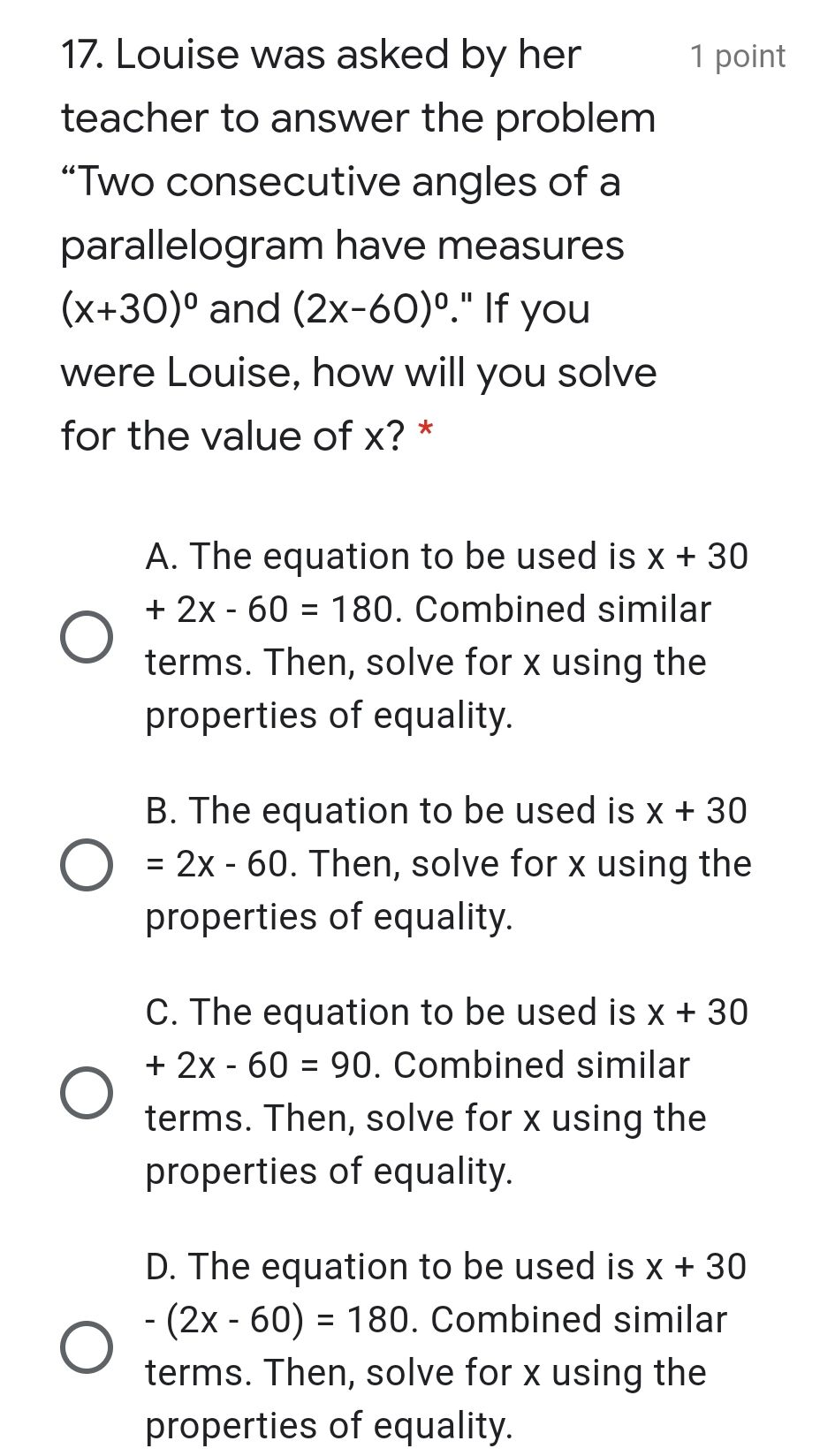### ¿Todavía tienes preguntas de matemáticas?

Pregunte a nuestros tutores expertos
Algebra
Pregunta17. Louise was asked by her teacher to answer the problem "Two consecutive angles of a parallelogram have measures $$x + 30$$ $$( x + 30 ) ^ { 0 }$$ and $$( 2 x - 60 ) ^ { 0 } . "$$ If you were Louise, how will you solve for the value of $$x ?$$ *

A. The equation to be used is $$x + 30$$ $$+ 2 x - 60 = 180$$ . Combined similar terms. Then, solve for $$x$$ using the properties of equality.

B. The equation to be used is $$x + 30$$ $$= 2 x - 60$$ . Then, solve for $$x$$ using the properties of equality.

C. The equation to be used is $$x + 30$$ $$+ 2 x - 60 = 90$$ . Combined similar terms. Then, solve for $$x$$ using the properties of equality.

D. The equation to be used is$$- ( 2 x - 60 ) = 180$$ . Combined similar terms. Then, solve for $$x$$ using the properties of equality.